Here we show some tests we performed to verify the correctness of the discrete packets discard in the hybrid router. See the implementation here

Summary
Original tests shows that the packets discards are less than the expected. Tracking the issue it apparently comes from the fact that E(pd/(Wc+Wd)) = E(pd) / E(Wc+Wd) where pd=PacketsDiscarded, Wc=continous packets, Wd=discreatePackets. Because of the distribution property for the multiplication of means it should be equal, but it is not!
But LOWERING the sampling rate (increasing samplingPeriod) of discrete packets (the one that senses discrete packets and outputs a continous rate) this effect diminishes. For example, if the sampling rate is set to 0.001 it results in less discrete discards than expected. When we set the samplingRate to 0.1, the discrete discards is corrected. This is counter intuitive as with bigger sampling period II would expect less presition.

The root explanation of the problem is still not understood completely. I need more knowledge in Statistics and SamplingI think it is very important to understand this effect to understand how to correctly set the samplingRate on each model. It probably depends on the rate of incoming packets. Intuitively I believe it should be small enough to provide good precition (whatever that means :-)). And apparently (I don't understand why) it should be big enough so that generated samples are different from each other.
Towsley has some paragraphs talking about the sampling rate in this model. They concluded that the sampligRate should

Also, another sampling method might be more effective.

The setting of the samplingRate greatly affects the execution performance (simulation times).

## Testing discards of discrete packets

### Test configuration

We configured the model as follows: capacity=1000Hz, Wc=1200Hz, Wd=500Hz.
Then 1200+500-1000=700 packets will be discarded in total per second. So the router will discard in total 41% of the packets. If the router is fair between the discards of the fluid and discrete flows, it should discard 500*0.41=200 of the discrete packets per second.

### Original Test results

It can be seen that as soon as the queue gets filled left) the rate at which discrete packets go out of the router dicreases because right) discards start apearing.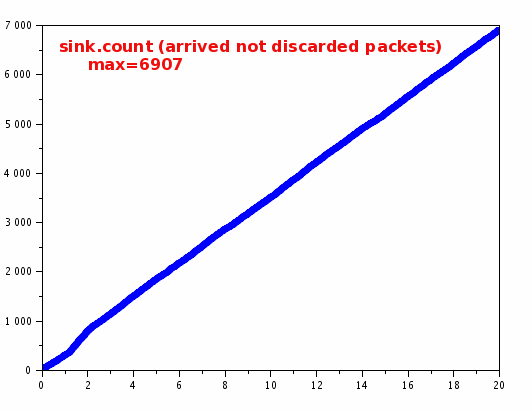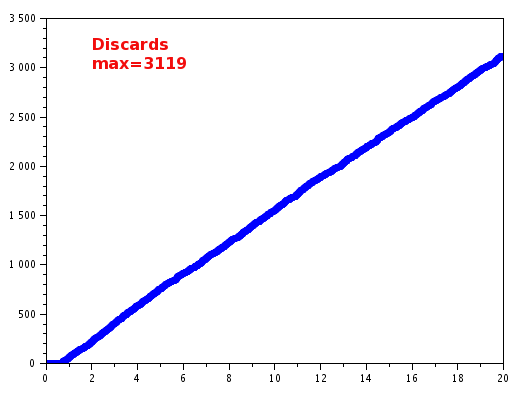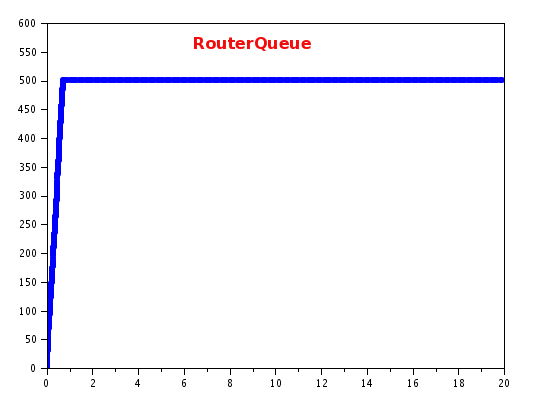### Validation (problems)

Nevertheless, there were less discrete packets discarded than expected. We were expecting ~200 packets to be discarded per second and we had 3119/20=155.95. Indeed, because for the first 0.75s there are no discards it would be more fair to calculate 3119/19=164.157, still not quite all right. 3119 packets represent the ~31% of the total packets (we were expecting 41% to be discarded).

Tracking the issue

To understand this fault we explore some values from the simulation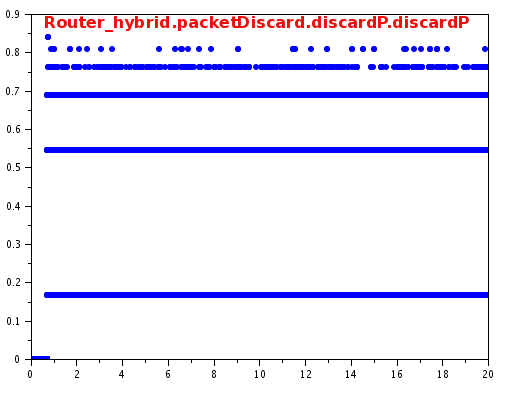So efectively, the packet discard model is discarding at the rate received. The problem then must come from the calculation of the rate.

mean(pd.value) = 701.545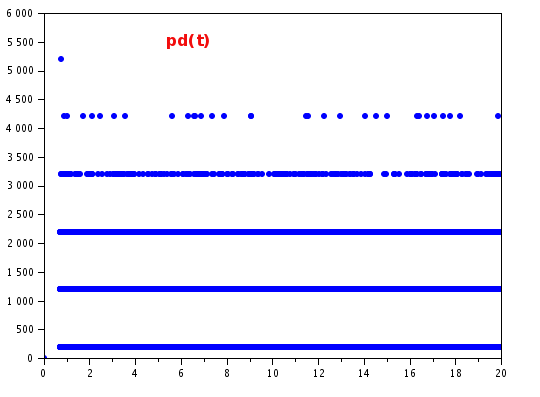So efectively the packet discard rate is as expected (with a mean of 700Hz). So the problem must come from the calculation

3) The sum of Wd+Wc
mean(Router_hybrid.sum.value) = 1701.55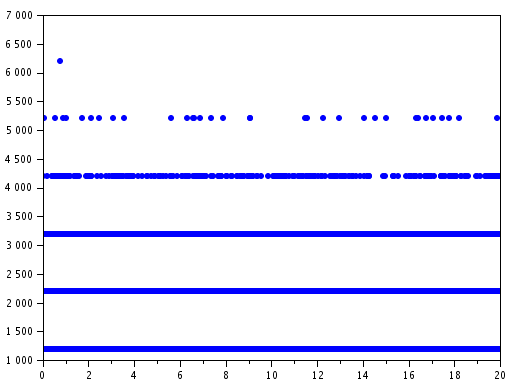So efectively the sum of both flows is as expected (with a mean of 1700Hz)

4) So the last piece to check is the NLFunction (pd/(Wc+Wd)

even if we don't take into account the first 0.75s, this calculation is still not OK: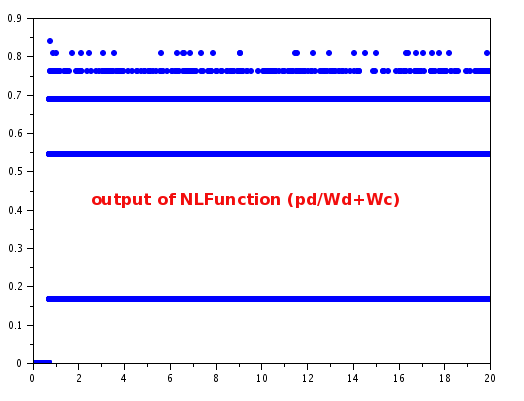We still need to understard why the NLFunction is receiving a signal u0=mean(700) and a signal u1=mean(1700) and it is outputing a signal which is calculated as u0/u1=mean(0.31). It is expected to be 700/1700 = 0.41.

I tried with executing several simulations and always the output values are similar, so it is not a problem of statistical significanse.

Something that attracts the attention is that all previous variables seems to be getting values in very well defined clusters. For example, the sum of Wd+Wc only gets the values: 1200, 2200,
3200, 4200, 5200 and 6200. Only those value, nothing in the middle.
This values in turn are the calculation of Wc+Wd. Wc is always constant 1000. Wd is calculated samping the arriving discrete packets.

If we plot the sampled Wd.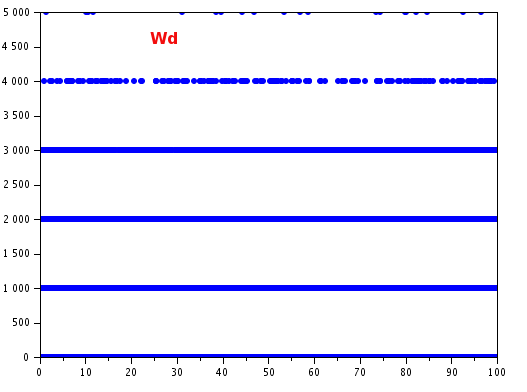This makes sense, as we have configured a sampling rate of 0.001s. The plot shows that in that period (0.001s) the amount of arrived packets was either 1, 2, 3, 4 or 5 packets.
This depends on the generation distribution, which was set to an exponential with mean value of 1/500 (it generates packets with a mean of 500 packets/s). Indeed the sampling is consistent with this as mean(wd) = 497.43497.

Although the mean value is correct it seems strange to see that the actual values are so well defined in clusters.

To change this fact, we reduced the sampling period. This will cause the model to generate less samples (less precition), but each sample will have different values.

### Increasing simulation time and repeating sevaral times

Same tests but increasing the total simulation time to 100s. This is to discard that it is not an effect of having few samples.

With more simulation time we observe the same defect: the number of discarded discrete packets is less than expected. We expected 41% of packets to be discarded, but only 32% were discarded.

There were 33680 packets which arrived to destination and 16280 that were discarded (total of 49960 packets, ~500Hz as configured). 16280 represents the 32.58 % of the total packets, and we were expecting 41%.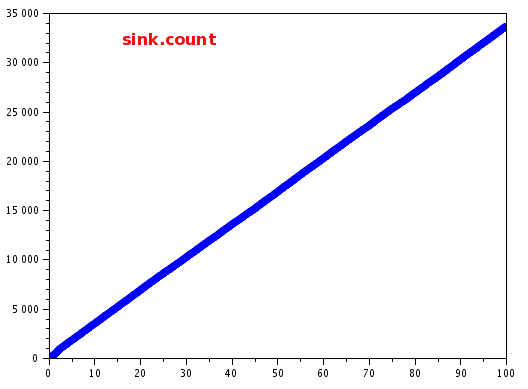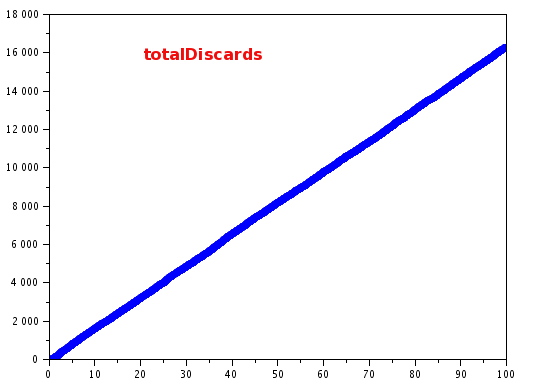Again, this 32.58% matches with the calculation of the DiscardProbability =pd / (Wc + Wd).

Redoing this same experiment (100s each simulation) a couple more times, it yielded the following percentages of discarded packets: 32.69050%, 32.69833%, 32,82305%, 32.57326%

### Changing samplingPeriod to 0.1s

Although the mean value is correct it seems strange to see that the actual values are so well defined in clusters. To change this fact, we reduced the sampling period. This will cause the model to generate less samples (less precition), but each sample will have different values.

We changed the parameter of the sampling rate to 0.1s.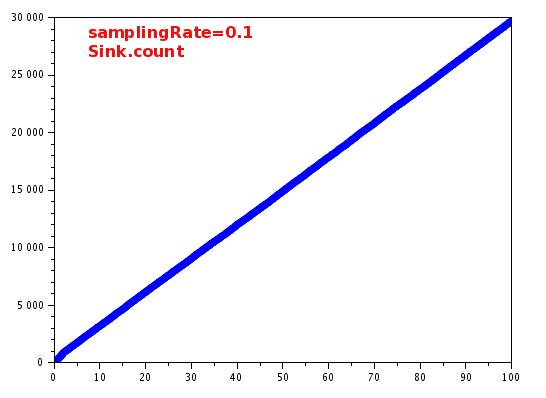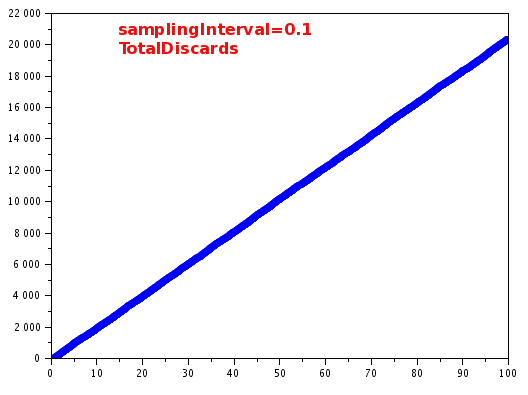Here we see that the amount of packets that arrived to destination is 29646 after 100s. The total discars is 20356. That means 40.7% of the packets were discarded. This is much closed to the expected value of 41%. Still there is a 1% discrepacy

If we now look at the discard probablily (discardP = pd / (Wc+Wd) ), we see that it takes in a bigger range of values. These are the values received as parameter by the packetDiscard model.
The mean value mean(Router_hybrid.saveDiscardRate.value)=0.4071131. This matches almost exactly to the percentage of packets discarded. But there is still a little difference with the expected value of 41%.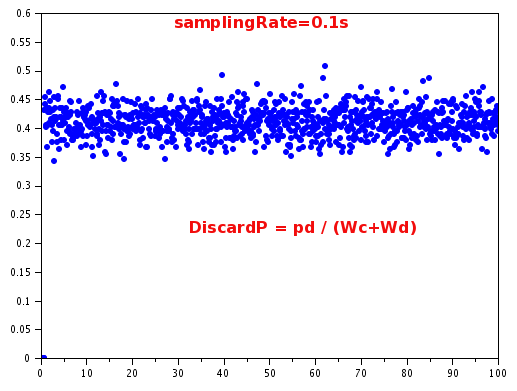discardP is calculated (based on pd and on (Wc+wd). Looking at these 2 values:

mean(Router_hybrid.pd.value) = 699.43662

mean(Router_hybrid.sum.value) = 1699.5305

699.43662 / 1699.5305 = 0.4115470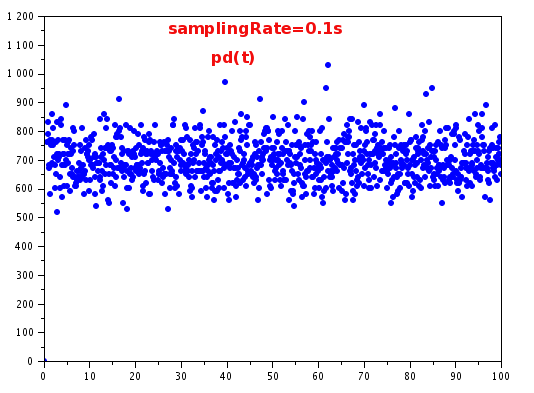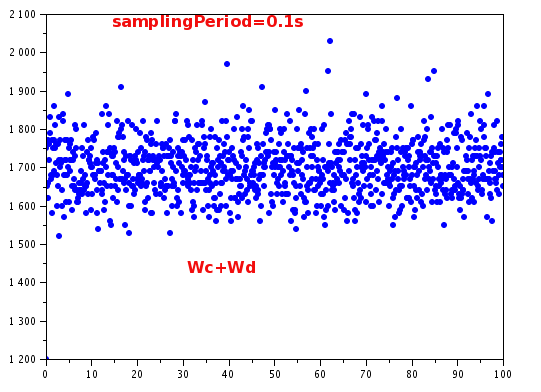So still the calculation of the DiscardP is failing. The E(pd/(Wc+Wd)) = E(pd) / E(Wc+Wd), which by properties of the mean it should be equal!

### Changing samplingPeriod to 1s

We performed the same experiment but increasing event more the samplingPeriod=1s. 100s of simulation. We obvserved much better relation between expected and measured value of discraded packets (error ~= 0.001). Nevertheless, the higher the samplingPeriod the model will adapt slower to changes in traffic flows (here it changes once per second, very low precition!).

We will pay attention to the following metrics:

DiscardProbability `mean(Router_hybrid.saveDiscardRate.value)         // The calculated discardProbability. Calculated as E[ pd/(Wc+Wd)]ExpectedDiscardRate=mean(Router_hybrid.u0.value) / mean(Router_hybrid.sum.value) // The expected discardProbability. Calculated as E[pd)] / E [Wc+Wd]totalDiscards=max(Router_hybrid.packetDiscard.totalDiscards.totalDiscards) // total discarded discrete packets totalArrived=max(PacketSink.count.count)                                                  // total arrived (not discarded) discrete packetspercentageDiscarded` totalDiscards / (totalDiscards+totalArrived) // percentage of discarded packets

Run 1
----------
totalArrived = 29658.

Run 2
-----------
totalArrived = 29672.

Run 3
---------
totalArrived = 29649.

## Pending things to try

1. Try setting the priority of the discard model to minimum. One possible explanation might be (just a guess): Imagine that we set the samplingPeriod really really small. Small enough so that always a maximum of one packet arrives in a samplingPerod. The output is always #sampledPackets/samplePeriod, so in this hipothetical that case the output of the sampler model would be always either 1/samplingPeriod or 0. If this happens when a packet arrives the discard model has last received a 0 discard probablity, so it will not discard the packet. Then it will receive a discard probability (if the samplingPeriod is very small, the sampler will output a very high value for a very short time). But this discard probability will last very little time as the sampler will output again 0 before any other packet arrives. Like this, no packets will be discarded at all. Maybe, what we are experiencing here (a discard rate lower than expected) is a similar sympthon but not so exagerated.
-- MatiasAlejandroBonaventura - 2016-08-12
Topic revision: r3 - 2016-08-15 - MatiasAlejandroBonaventuraLog In

Webs

Welcome GuestCern SearchTWiki SearchGoogle Search Main All websCopyright &© 2008-2019 by the contributing authors. All material on this collaboration platform is the property of the contributing authors.
Ideas, requests, problems regarding TWiki? Send feedback